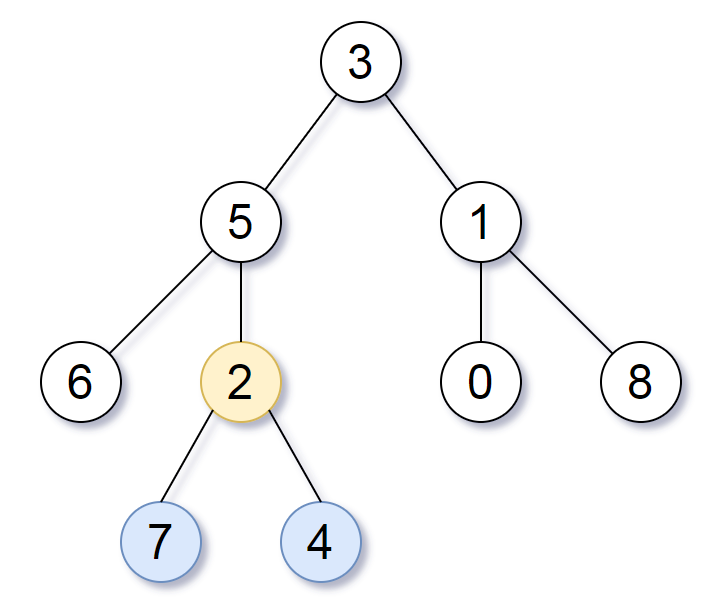1123. Lowest Common Ancestor of Deepest Leaves
Medium
1.7K
793

Given the `root` of a binary tree, return the lowest common ancestor of its deepest leaves.

Recall that:

• The node of a binary tree is a leaf if and only if it has no children
• The depth of the root of the tree is `0`. if the depth of a node is `d`, the depth of each of its children is `d + 1`.
• The lowest common ancestor of a set `S` of nodes, is the node `A` with the largest depth such that every node in `S` is in the subtree with root `A`.

Example 1:```Input: root = [3,5,1,6,2,0,8,null,null,7,4]
Output: [2,7,4]
Explanation: We return the node with value 2, colored in yellow in the diagram.
The nodes coloured in blue are the deepest leaf-nodes of the tree.
Note that nodes 6, 0, and 8 are also leaf nodes, but the depth of them is 2, but the depth of nodes 7 and 4 is 3.```

Example 2:

```Input: root = 
Output: 
Explanation: The root is the deepest node in the tree, and it's the lca of itself.
```

Example 3:

```Input: root = [0,1,3,null,2]
Output: 
Explanation: The deepest leaf node in the tree is 2, the lca of one node is itself.
```

Constraints:

• The number of nodes in the tree will be in the range `[1, 1000]`.
• `0 <= Node.val <= 1000`
• The values of the nodes in the tree are unique.

Note: This question is the same as 865: https://leetcode.com/problems/smallest-subtree-with-all-the-deepest-nodes/

Accepted
91.4K
Submissions
128.9K
Acceptance Rate
70.9%

Seen this question in a real interview before?
1/4
Yes
No

Discussion (0)

Related Topics